# OECD Doctors' consultations data analysis 6 - using lm() function for linear regressionPhoto by DAVID TANG on Unsplash

This post is following of above post.

In this blog, I will do regression anaysis using lm() function in R.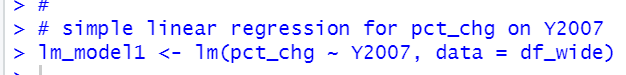summary() function displays result.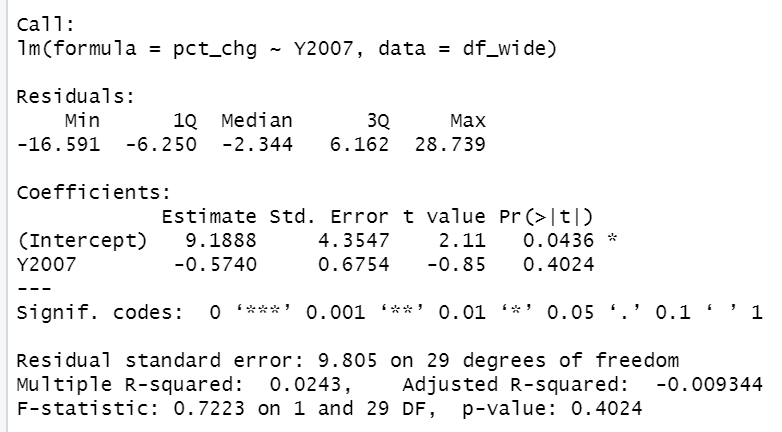p-value is 0.4024, it is greater than 0.05, so this model is not valid model.

Let's add 'region' to explanatory variable.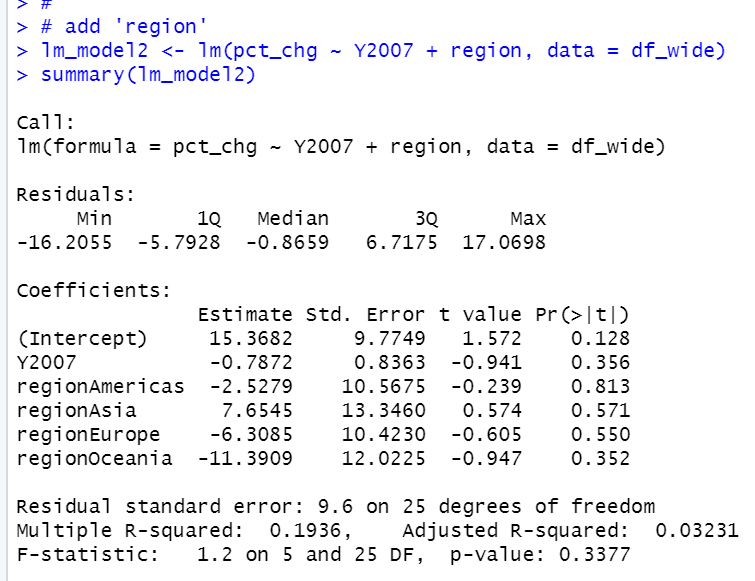p-value is 0.3377.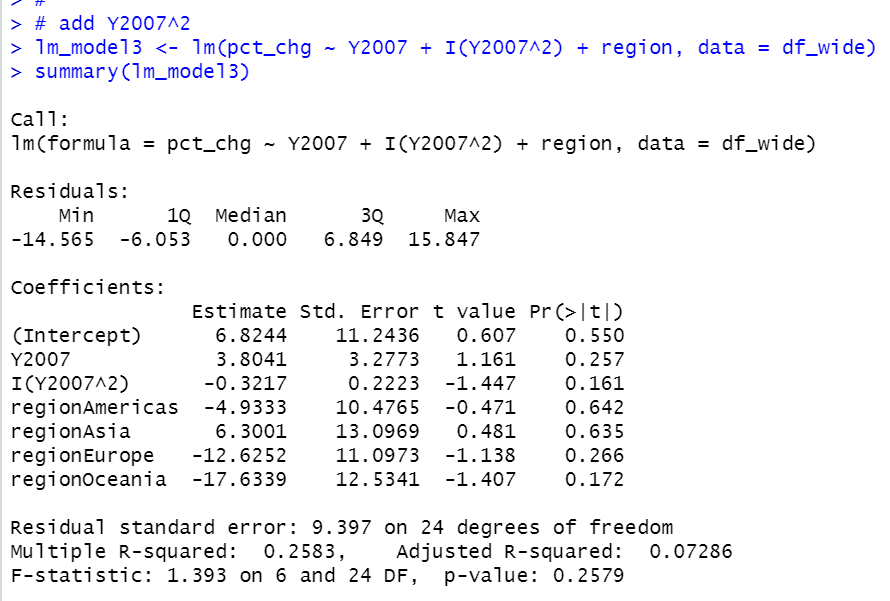p-value is 0.2579, it is not valid.

Uhmm.... let's add Y2007^3 , Y2007^4 and Y2007^5.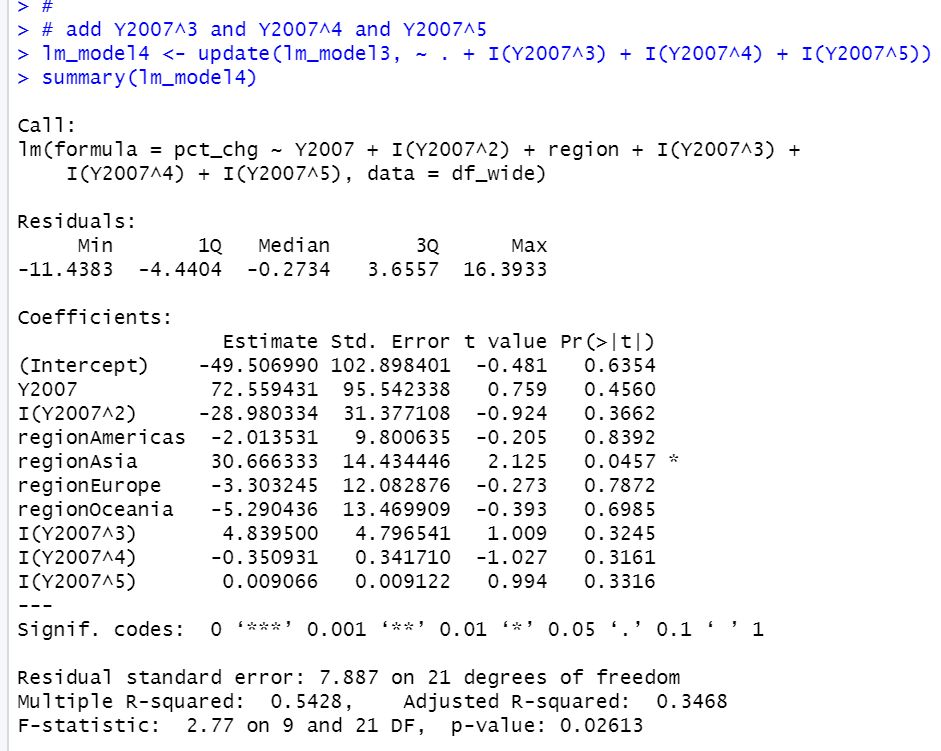p-value is o.02613, it is smaller than 0.05.

Let's plot scatther plot of Y2007 and pct_chg and add predicted values.

First, makeing plot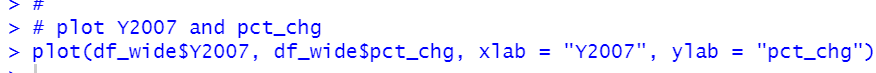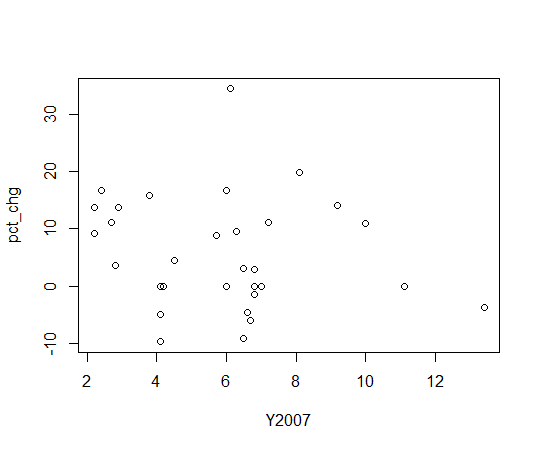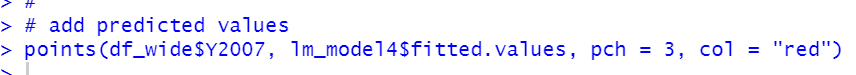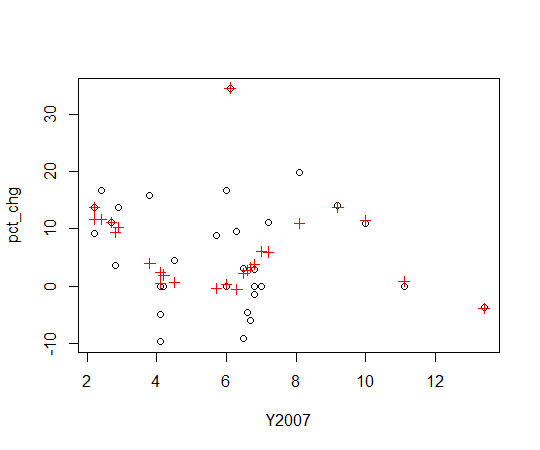red + is predicted value by lm_model4.

That's it. Thank you!